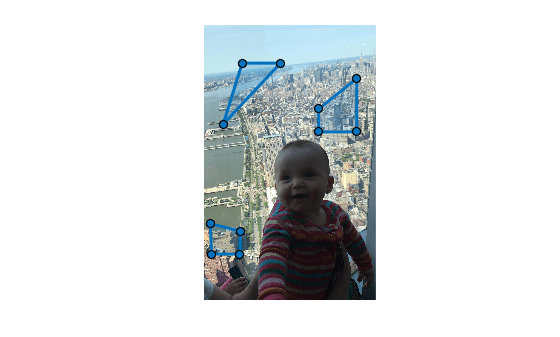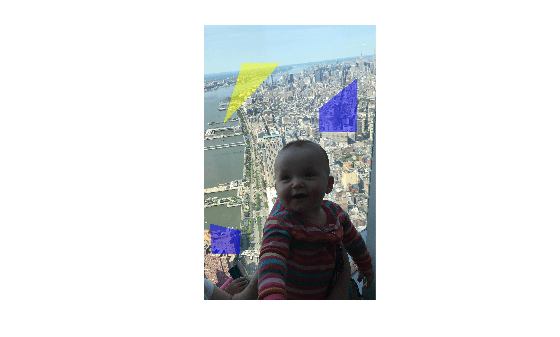# poly2label

Create label matrix from set of ROIs

Since R2020b

## Syntax

``L = poly2label(roiPositions,roiLabelIDs,imageSize)``
``L = poly2label(roiPositions,roiLabelIDs,R)``

## Description

example

````L = poly2label(roiPositions,roiLabelIDs,imageSize)` creates a numeric label matrix `L` from the regions of interest (ROIs) defined in `roiPositions`. `roiLabelIDs` specifies the numeric ID for each ROI in `roiPositions`. `imageSize` specifies the size of the output label matrix.```
````L = poly2label(roiPositions,roiLabelIDs,R)` creates a numeric label matrix where the spatial referencing object `R` specifies the coordinate system used by the ROI positions in `roiPositions`. The function assumes that the ROI positions are in world limits defined by `R`. The `ImageSize` property of `R` specifies the size of the label matrix `L`.```

## Examples

collapse all

Read an image into the workspace and display it.

``` figure I = imread('baby.jpg'); imshow(I)```

Initialize the ROI position cell array and image size variables. If you pass `poly2label` a size value containing three dimensions, it only uses the first two, m-by-n.

```numPolygon = 3; roiPositions = cell(numPolygon,1); imSize = size(I);```

Specify the coordinates of three ROIs in the `roiPositions` cell array. In this example, the first ROI is a triangle, requiring coordinates for three corners. The other two ROIs are quadrilaterals, requiring coordinates for four corners.

```roiPositions{1} = [500 500; 250 1300; 1000 500]; roiPositions{2} = [1500 1100; 1500 1400; 2000 1400; 2000 700]; roiPositions{3} = [80 2600; 480 2700; 470 3000; 100 3000];```

Create an array for label IDs the same size as the `roiPositions` cell array.

`roilabelID = zeros(numPolygon,1,'uint8');`

Specify label ID values that correspond to the order in which you listed the ROIs in `roiPositions`. The first ROI is a triangle so give it the label `1`. The next two ROIs are both quadrilaterals so give them the label `2`.

```roilabelID(1) = 1; roilabelID(2) = 2; roilabelID(3) = 2;```

Draw the three ROIs on the figure.

```for id = 1:numPolygon drawpolygon('Position',roiPositions{id}); end```Create a label matrix from the ROIs. The label matrix is the same size, m-by-n, as the original image.

`L = poly2label(roiPositions,roilabelID,imSize);`

Display the label matrix overlaid on the original image.

```figure; B = labeloverlay(I,L); imshow(B);```## Input Arguments

collapse all

Coordinate vectors, specified as a 1-by-P cell array of numeric vectors, where P is the total number of ROIs. Each cell array element is an s-by-2 coordinate vector of the form ```[x1 y1; …; xs ys]```, where s is the total number of vertices for that ROI. Each x,y pair defines a vertex of the ROI. If the ROI shape is not already closed, the `poly2label` function closes the shape automatically. You can specify any number of ROIs.

Data Types: `double` | `cell`

Labels for each ROI, specified as a numeric vector of the same length as the `roiPositions` argument. Each label in the vector corresponds to the ROI in the associated position in the `roiPositions` cell array.

`poly2label` assigns the value `0` to all background pixels in the output image.

Data Types: `single` | `double` | `int8` | `int16` | `int32` | `uint8` | `uint16` | `uint32`

Size of the output label matrix, specified as a 2- or 3-element numeric vector. If you specify a 3-element vector, the `poly2label` function uses only the first two dimensions, m-by-n.

Data Types: `double`

Spatial referencing information, specified as an `imref2d` object.

## Output Arguments

collapse all

Label matrix, returned as an m-by-n matrix of nonnegative values of the same data type as `roiLabelIDs`. Pixels labeled `0` are the background.

## Tips

• The `poly2label` function sets pixels that are inside an ROI to a label value. For more information about classifying pixels on the ROI boundary, see Classify Pixels That Are Partially Enclosed by ROI.

• When the positions of several ROIs overlap each other, the ROI label with the lowest index number in the `roiPositions` cell array overwrites the other ROIs.

## Version History

Introduced in R2020b